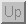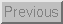Next: 3.2 Force Sensing Resistors Up: 3 Sensors Previous: 3 Sensors

## 3.1 Piezoelectric Sensors

The Piezoelectric effect is an effect in which energy is converted between mechanical and electrical forms. It was discovered in the 1880's by the Curie brothers. Specifically, when a pressure (piezo means pressure in Greek) is applied to a polarized crystal, the resulting mechanical deformation results in an electrical charge. Piezoelectric microphones serve as a good example of this phenomenon. Microphones turn an acoustical pressure into a voltage. Alternatively, when an electrical charge is applied to a polarized crystal, the crystal undergoes a mechanical deformation which can in turn create an acoustical pressure. An example of this can be seen in piezoelectric speakers. (These are the cause of those annoying system beeps that are all too common in today's computers).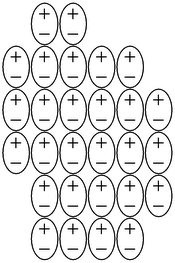Figure 3: Internal Structure of an electret

Electrets are solids which have a permanent electrical polarization. (These are basically the electrical analogs of magnets, which exhibit a permanent magnetic polarization). Figure 3 shows a diagram of the internal structure of a electret. In general, the alignment of the internal electric dipoles would result in a charge which would be observable on the surface of the solid. In practice, this small charge is quickly dissipated by free charges from the surrounding atmosphere which are attracted by the surface charges. Electrets are commonly used in microphones.

Permanent polarization as in the case of the electrets is also observed in crystals. In these structures, each cell of the crystal has an electric dipole, and the cells are oriented such that the electric dipoles are aligned. Again, this results in excess surface charge which attracts free charges from the surrounding atmosphere making the crystal electrically neutral. If a sufficient force is applied to the piezoelectric crystal, a deformation will take place. This deformation disrupts the orientation of the electrical dipoles and creates a situation in which the charge is not completely canceled. This results in a temporary excess of surface charge, which subsequently is manifested as a voltage which is developed across the crystal.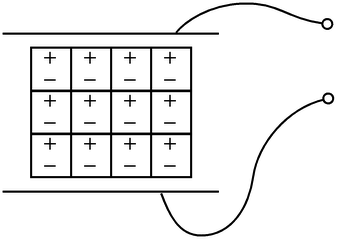Figure 4: A sensor based on the piezoelectric effect

In order to utilize this physical principle to make a sensor to measure force, we must be able to measure the surface charge on the crystal. Figure 4 shows a common method of using a piezoelectric crystal to make a force sensor. Two metal plates are used to sandwich the crystal making a capacitor. As mentioned previously, an external force cause a deformation of the crystal results in a charge which is a function of the applied force. In its operating region, a greater force will result in more surface charge. This charge results in a voltage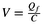, where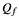is the charge resulting from a force f, and C is the capacitance of the device.

In the manner described above, piezoelectric crystals act as transducers which turn force, or mechanical stress into electrical charge which in turn can be converted into a voltage. Alternatively, if one was to apply a voltage to the plates of the system described above, the resultant electric field would cause the internal electric dipoles to re-align which would cause a deformation of the material. An example of this is the fact that piezoelectric transducers find use both as speakers (voltage to mechanical) and microphones (mechanical to electrical).Next: 3.2 Force Sensing Resistors Up: 3 Sensors Previous: 3 Sensors

Tim Stilson
Thu Oct 17 16:32:33 PDT 1996